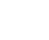# 数松果北师大二年级上册数学教师公开课PPT课件<p>数松果北师大版小学数学二年级上册教师公开课课件1.结合“数松果”的具体情境，经历5的乘法口诀的编制过程。2、理解5的乘法口诀，会用5的乘法口诀进行计算和解决简单的实际问题。学习目标：有几堆有几个15210315420525630735840945+5+5+5+5+5+5+5+51X5=52X5=103X5=154X5=205X5=255X6=305X7=355X8=405X9=451X5=52X5=103X5=154X5=205X5=255X6=305X7=355X8=405X9=45555555555一二三四五六七八九五五五五五五五五五五一十十五二十二十五三十三十五四十四十五得乘法口诀在我国有悠久的历史，早在两千多年前，我国古代劳动人民为了方便计算乘法，就编出了乘法口诀，它读起来顺口，所以就一代一代传承下来。这是我国独有的文化瑰宝，因此乘法口诀都是用汉字书写的。我说你答老师说几只手,你就喊出相应5的乘法口诀。生...

• 页数：32页
• 大小：19.57 MB
• 编号：10101
• 类型：VIP模板
• 格式：pptx
• 提示：数字产品不支持退货